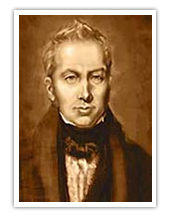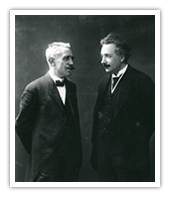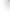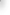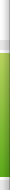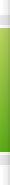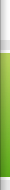# On the edge of chaos

How can we describe a complex entity?A complex should be described using statistical methods rather than with a deterministic approach. Then modelling is the answer to our question but there are many difficulties....

Complexity refers to situations where many simple interacting parts produce a collective, often unexpected, behaviour. The components of the system can self-organize in stable (in a statistical sense) state and can acquire collective properties which are not necessarily characteristic of each single component. Different parts of the complex behave differently but these different parts are not indipendent in the system. Therefore there is a basic duality between parts which are at the same time distinct and connected.
The aspect of distinction leads to the concept of variety, heterogeneity, disorder, chaos and entropy; on the other hand connection corresponds constraint, redundancy, order and negentropy. Complexity lies between these two aspects: neither perfect disorder (which can be described statistically) nor perfect order (which can be described by deterministic methods) .... just “on the edge of chaos”.
The simple way to model order is through the concept of symmetry; in symmetric patterns one part of the system is sufficient to recontruct the whole. But disorder too is characterized by symmetry! Not of the actual position of the component but of the probabilities that a component can be found at a particular position.Intuitively complexity can be characterized by a lack of symmetry by the fact that no part or aspect of a complex system can provide sufficient information to actually or statistically predict the properties of the other parts. These aspects show clearly the difficulties inmodelling a complex entity.

# When did complexity become a science?

Historically and philosophically the concept of complexity is rather recent. The natural philosophers in ancient Greece were focused on the quest of the first principle (arkè) from which everythings had to descend. Then the scientific thought began its progress to the modern classic physics of Galileo and Newton. Hereafter the fundamental concept was determinism but the developments of natural sciences in the nineteenth century open new scenarios and deterministic approach loose partly its head role. The new aim of science describing the natural phenomena as stochastic processes and modelling them.

# From pollen’s grains to financial markets

In 1827 the botanist Robert Brown observed the behaviour of pollen’s grains suspanded in a water solution: the grains moved in a chaotic way, following random trajectories. This motion, after just called Brownian motion, is due to the collisions between the molecules of the fluids and the particles suspanded in it. In 1905 Albert Einstein gave a theoric interpretation of the phenomenon. His theory was based on the observation of the motion from a microscopic point of view and on the other hand from the macroscopic one. Infact the unknoledge of the initial conditions leads to the necessity of analyze macroscopically the system in order to model it. In practical terms the microscopic motion of the molecules and theparticles is so complicated that the only way to describe it is by means of statistical methods.
Einstein’s work is fundamental because represents the first application of stochastic models to natural phenomena. Not long after another famous scientist obtained the same results with a simplest method: Langevin proposed to resolve a differential equation, the Langevin equation, whose solution is a random variable. The equation describes the temporal evolution of the so called Markovian stochastic processes and represents the basis of several modern models applied in very different disciplines. An example is given by turbulent dispersion models for tracers (pollutants) in the atmosphere which simulate the effects of turbulent eddies considering the particle fluid velocity as a stochastic variable described by a proper Langevin equation.

Another important contribution to this type of studies came from Louis Bachelier . In 1901, some years before Einstein, he analyzed the temporal variations of the prices of state bonds and found that they had a behaviour similar to that of the grains of Brown. E proposed the first macroscopic model for financial markets and defined a stochastic process, better known as the Wiener model, directly connected to the diffusion equation. It was born econophysics, another of the several diciplines that come into the vaste area of complexity.